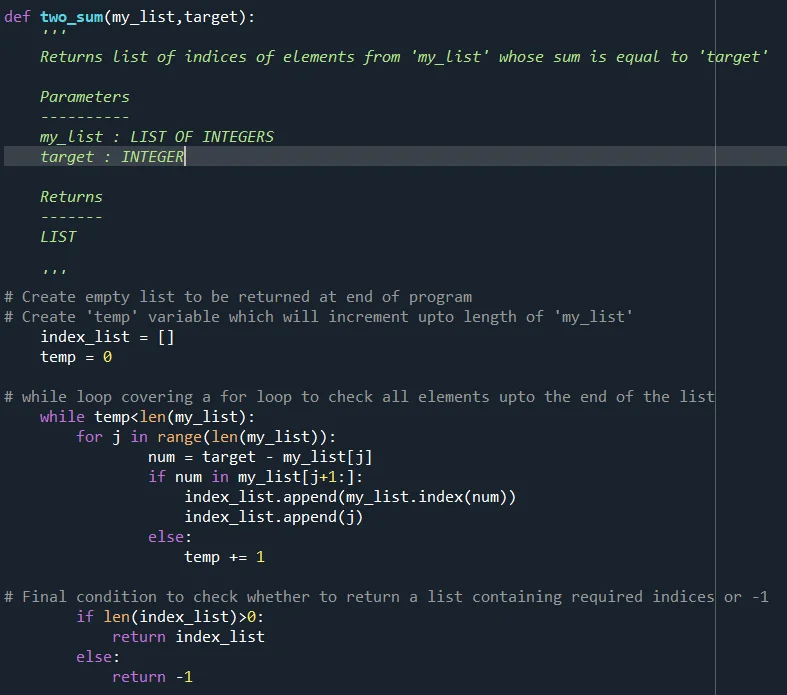Posted on:

10 May 2023

0

# two sum solution with more than one pairs satisfying the requirement

The program shown in the course is relevant only for the 1st pair from the list. However, if there are more than one such pairs, this program does not work.

I would like to propose a program which covers the two sum solution even if there are more than one pairs satisfying the target value as below. I tried it for all possibilities and it workd fine. The text of the program is reproduced below:

def two_sum(my_list,target):
'''
Returns list of indices of elements from 'my_list' whose sum is equal to 'target'

Parameters
----------
my_list : LIST OF INTEGERS
target : INTEGER

Returns
-------
LIST

'''
# Create empty list to be returned at end of program
# Create 'temp' variable which will increment upto length of 'my_list'
index_list = []
temp = 0

# while loop covering a for loop to check all elements upto the end of the list
while temp<len(my_list):
for j in range(len(my_list)):
num = target - my_list[j]
if num in my_list[j+1:]:
index_list.append(my_list.index(num))
index_list.append(j)
else:
temp += 1

# Final condition to check whether to return a list containing required indices or -1
if len(index_list)>0:
return index_list
else:
return -1

Suggestion: take the list as [1,2,3,4,5] and target as 5